# RS Aggarwal Class 7 Solutions Chapter 10 Percentage Ex 10B

## RS Aggarwal Class 7 Solutions Chapter 10 Percentage Ex 10B

These Solutions are part of RS Aggarwal Solutions Class 7. Here we have given RS Aggarwal Solutions Class 7 Chapter 10 Percentage Ex 10B.

Other Exercises

Question 1.
Solution:
Rupesh seemed 495 marks out of 750
Percentage of marks = $$\frac { 495 }{ 750 }$$ x 100 = 66%

Question 2.
Solution:
Monthly salary = Rs. 15625
Increase = 12%
Amount of increase = $$\frac { 15625 x 12 }{ 100 }$$ = Rs. 1875
New salary = Rs. 15625 + Rs. 1875 = Rs. 17500

Question 3.
Solution:
Excise duty in the beginning = Rs. 950
Reduced duty = Rs. 760
Reduction = Rs. 950 – Rs. 760 = Rs. 190
Reduction percent = $$\frac { 190 x 100 }{ 950 }$$ = 20%

Question 4.
Solution:
Let x be the total cost of the T.V
96% of x = 10464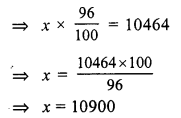Total cost of T.V = Rs. 10900

Question 5.
Solution:
Let number of students = x
In a school boys = 70%
girls = 100 – 70 = 30%
Now 30% of x = 504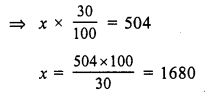Number of boys = 1680 – 504 = 1176
and number of total students = 1680

Question 6.
Solution:
Copper required = 69 kg
copper in ore = 12%
Let quantity of ore = x kg
12% of x = 69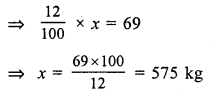Quantity of ore = 575kg

Question 7.
Solution:
Pass marks = 36%
A students gets marks = 123
But failed by 39 marks
Pass marks = 123 + 39 = 162
Now, 36% of maximum marks = 162
Maximum marks = $$\frac { 162 x 100 }{ 36 }$$ = 450 marks

Question 8.
Solution:
Let number of apples = x
Number of apples sold = 40% of x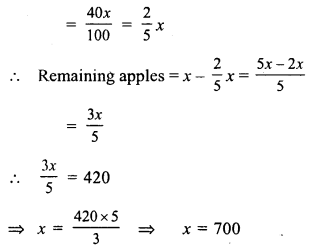Hence number of apples = 700

Question 9.
Solution:
Let total number of examinees = x
the numbers of examinees who passed = 72% of x
= $$\frac { x x 72 }{ 100 }$$
= $$\frac { 18x }{ 25 }$$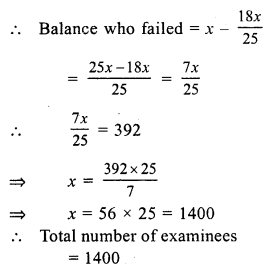Question 10.
Solution:
Let the gross value of moped = x
Amount of commission = 5% of x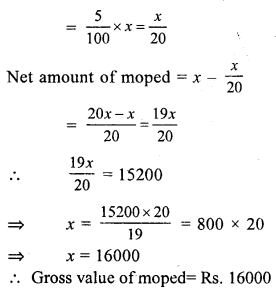Question 11.
Solution:
Total gunpowder = 8 kg
Amount of nitre = 75%
amount of sulphur = 10%
Rest of powder which is charcoal = 100 – (75 + 10) = 100 – 85 = 15 = 15%
Amount of charcoal = 8 x $$\frac { 15 }{ 100 }$$ = $$\frac { 120 }{ 100 }$$
= $$\frac { 6 }{ 5 }$$ kg = 1 kg 200 grams = 1.2 kg

Question 12.
Solution:
Quantity of chalk = 1 kg or 1000 g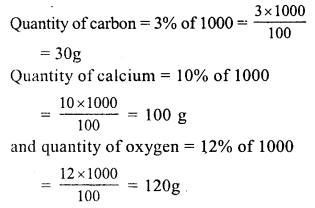Question 13.
Solution:
Let total number of days on which the
school open = x
and Sonal’s attendance = 75%
x x 75% = 219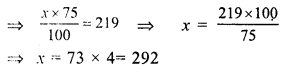No. of days on which was school open = 292 days

Question 14.
Solution:
Rate of commission = 3%
Amount of commission = Rs. 42660
Let value of property = x
then 3% of x = 42660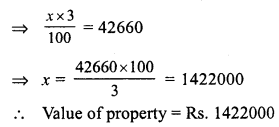Question 15.
Solution:
Total votes of the constituency = 60000
= $$\frac { 80 }{ 100 }$$ x 60000 = 48000
= $$\frac { 60 }{ 100 }$$ x 48000 = 28800
Votes polled in favour of B = 48000 – 28800 = 19200

Question 16.
Solution:
Let original price of shirt = Rs. x
Discount = 12%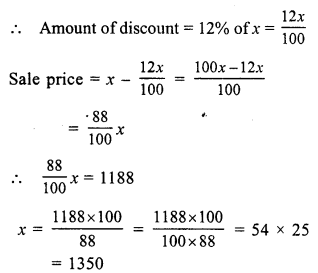Original price of shirt = Rs. 1350

Question 17.
Solution:
Let original price of sweater = x
Rate of increase = 8%
Increased price = x + 8% of x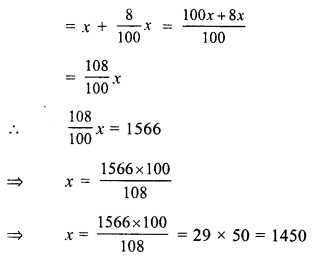Hence, original price of sweater = Rs. 1450

Question 18.
Solution:
Let total income = x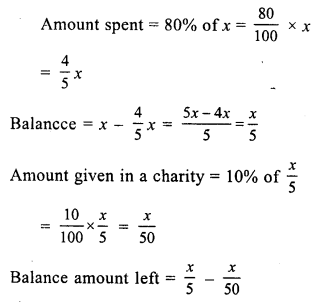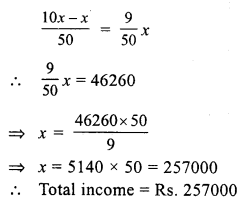Question 19.
Solution:
Let the given number = 100
Then increase % = 20%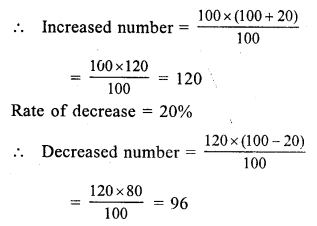Decrease = 100 – 96 = 4
Decrease per cent = 4%

Question 20.
Solution:
Let original salary of the officer = Rs. 100
Increase = 20%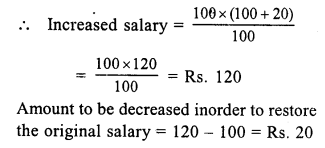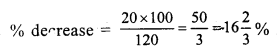Question 21.
Solution:
Rate of commission = 2% on first Rs. 200000
1 % on next Rs. 200000 and 0.5% on remaining price
Sale price of property = Rs.200000 + 200000 +140000 = Rs. 540000
Now commission earned by the
= Rs. 200000 x 2% + Rs. 200000 x 1% + 140000 x 0.5%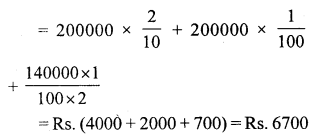Question 22.
Solution:
Let Akhil’s income = Rs. 100
Then income of Nikhil’s will be = Rs. 100 – 20 = Rs. 80
Amount which is more than that of Akhil’s = 100 – 80 = Rs. 20
% age = $$\frac { 20 x 100 }{ 80 }$$ = 25%

Question 23.
Solution:
Let income of Mr Thomas = Rs. 100
then income of John = Rs. 100 + 20 = Rs. 120
Income of Mr Thomas is less than John = Rs. 120 – 100 = Rs. 20
% age = $$\frac { 20 x 100 }{ 120 }$$
= $$\frac { 50 }{ 3 }$$ = 16$$\frac { 2 }{ 3 }$$ %

Question 24.
Solution:
Present value of machine = Rs. 387000
Rate of depreciation = 10%
Let 1 year ago the value of machine was = x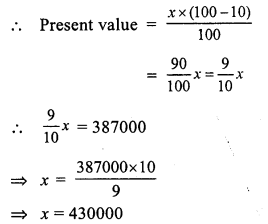1 year ago, value of machine = Rs. 430000

Question 25.
Solution:
Present value of car = Rs. 450000
Rate of decreasing of value = 20%
Value after 2 years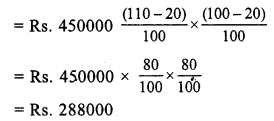Question 26.
Solution:
Present population = 60000
Rate of increase = 10%
Increased population after 2 years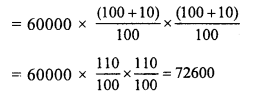Question 27.
Solution:
Let the price of sugar = Rs. 100
and consumption = 100 kg.
Increase price of 100 kg = Rs. 100 + 25 = Rs. 125
Now increased amount on 100 kg = Rs. 125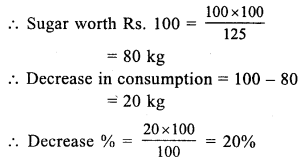Hope given RS Aggarwal Solutions Class 7 Chapter 10 Percentage Ex 10B are helpful to complete your math homework.

If you have any doubts, please comment below. Learn Insta try to provide online math tutoring for you.